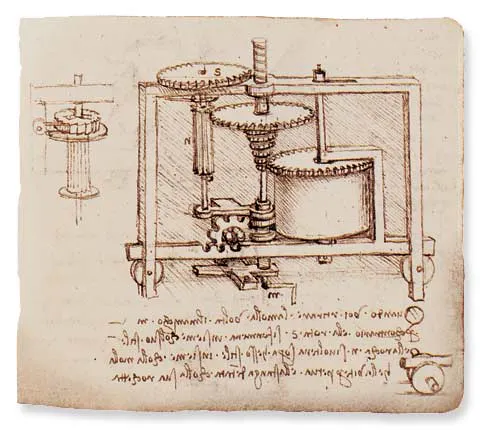# Industrial Energy Consumption Console

This is an industrial class console constructed to help in the data collection of industrial process.

BeginnerFull instructions provided4 hours871## Things used in this project

### Hardware components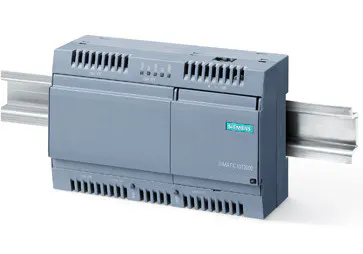Siemens SIMATIC IOT2020
×1
 arduino proto shield
×1
 Omron G2R-1-S(S) SPDT Relay
×6
 4n35 optocoupler
×4LED (generic)
×4Resistor 10k ohm
×4Resistor 1k ohm
×4Jumper wires (generic)
×1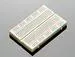×1
×1

### Software apps and online servicesArduino IDEUbidots

## Custom parts and enclosures

### Cover proposal

Here a design for a cover, the center of this design is a small pcb with a pcb screw terminal block, this pcb also includes a 4 pin female header so you can get the security of a bolted connector for your input signals and the facility to connect this with the iot2020 board with standard male jumpers.
Now we know that siemens has released an IO Shield for the IOT2000 (article number 6ES7647-0KA01-0AA2), with this you can put aside the cover and the rest of the components mounted on the breadboard.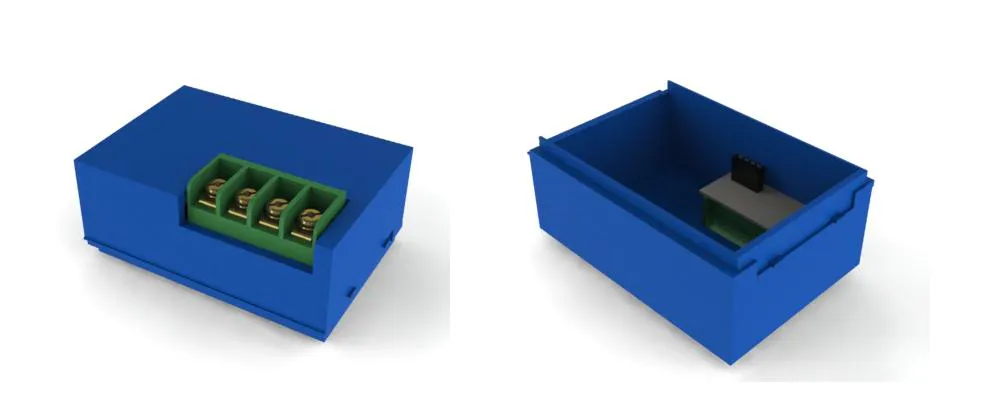## Schematics

### Signals

The input signals to our console will come from the current electrical system, in the case of the loading spouts the signal of 24v will be taken from the solenoid switch PB2 of the discharge valve of the silo, in the case of the vibrating screen, the signal of 24v will come from the motor contactor switch.
With all the above we will have 3 input signals (output of a OR gate made by relays) from retractile sleeves and an input signal from the vibrating screen, this gives us 4 signals: I1 I2, I3 and I4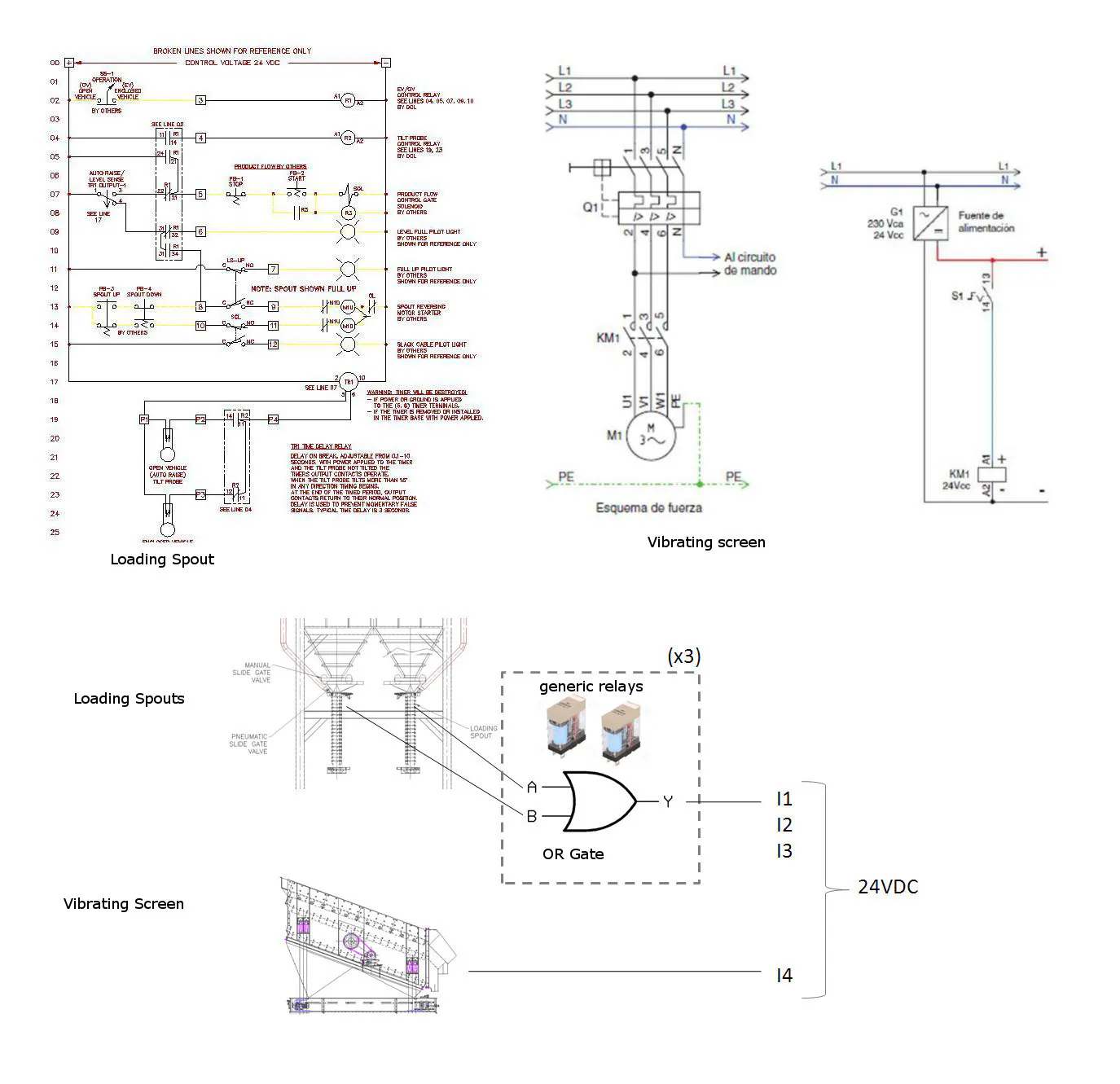### Relays

Schematic of the OR gates made with relays, in this case 6 generic SPDT relays from omron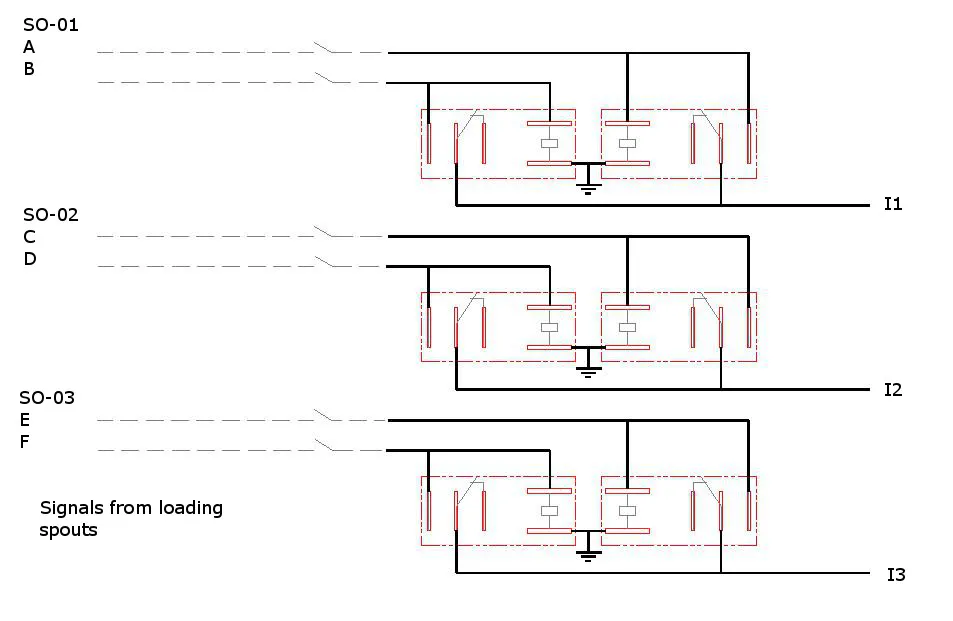### Protoboard

Here the schematic of the protoboard with the components, important note: the 1K resistor must be of 1 watt.
For more inputs you can change the optocoupler with one with more channels.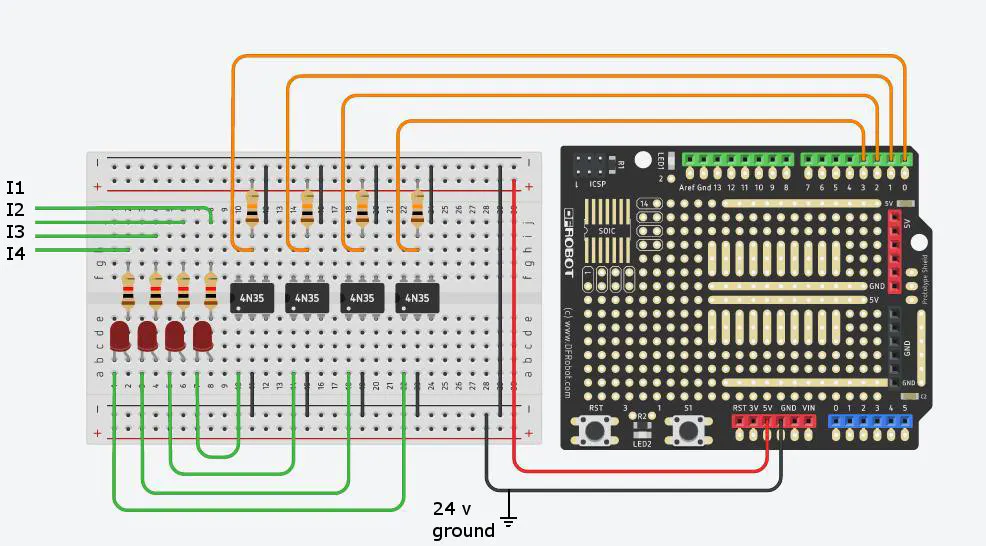### IOT2020 setup

Prior to work with arduino IDE and IOT2020 you need to follow the steps of this post: https://www.rs-online.com/designspark/program-the-iot2000-with-arduino-ide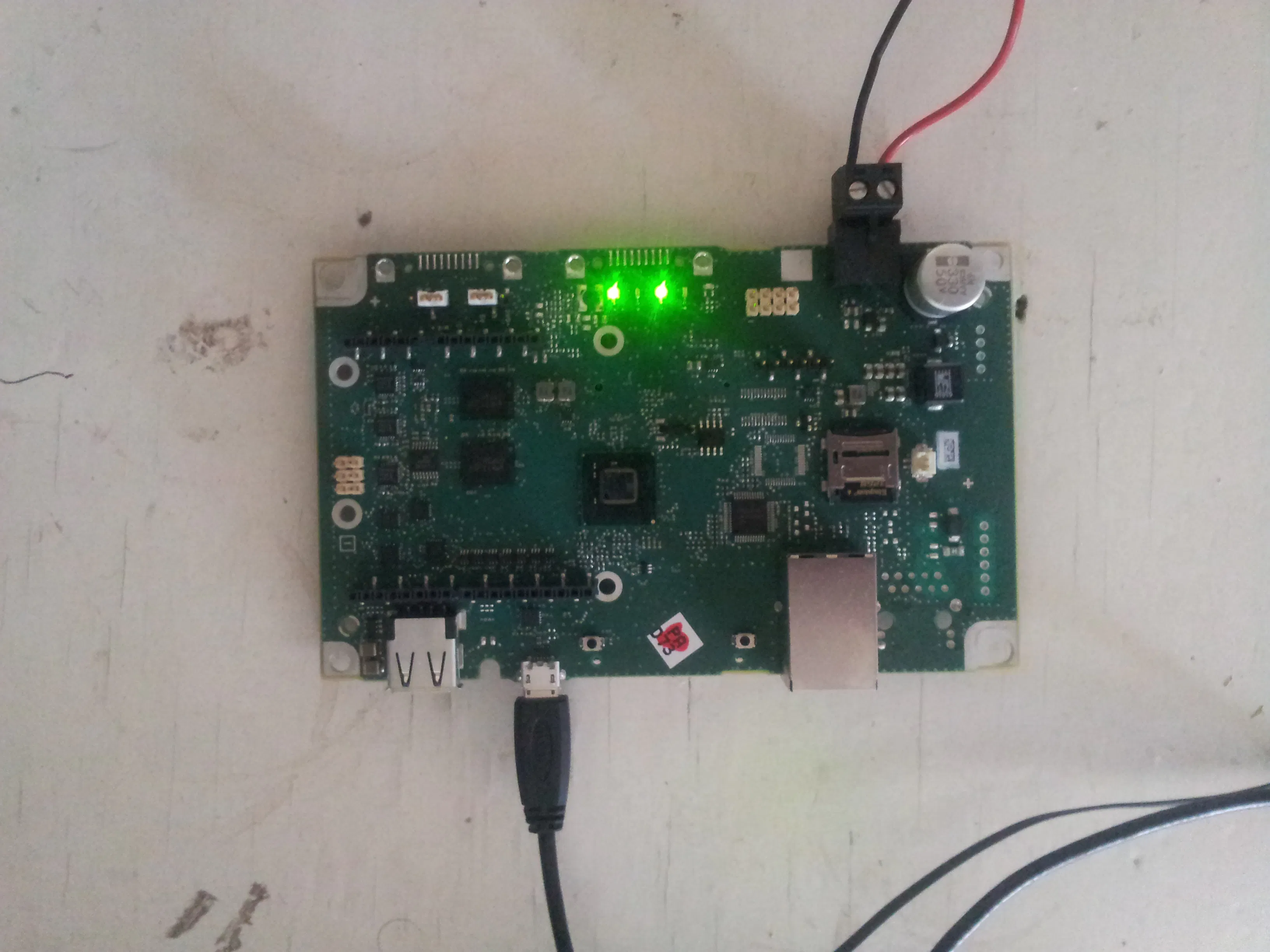### Ubidots setup

For the data collection i've selected Ubidots IoT platform, for start you need to do the next:
1.- Create an account
2.- In sources create a new data source
3.- Copy the label of your device an paste into the code
4.- From API credentials copy the token and paste into the code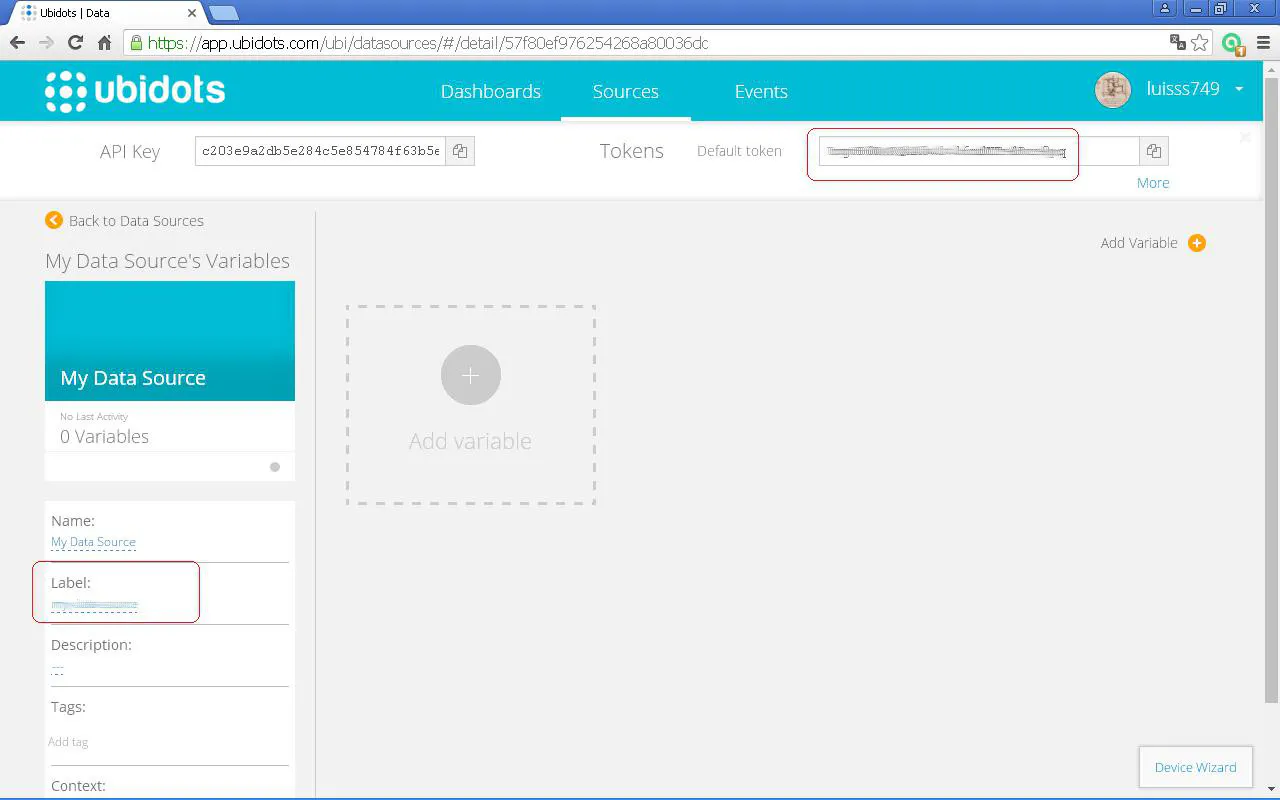### Ubidots device panel

Here you can see the behavior of the variables in real time.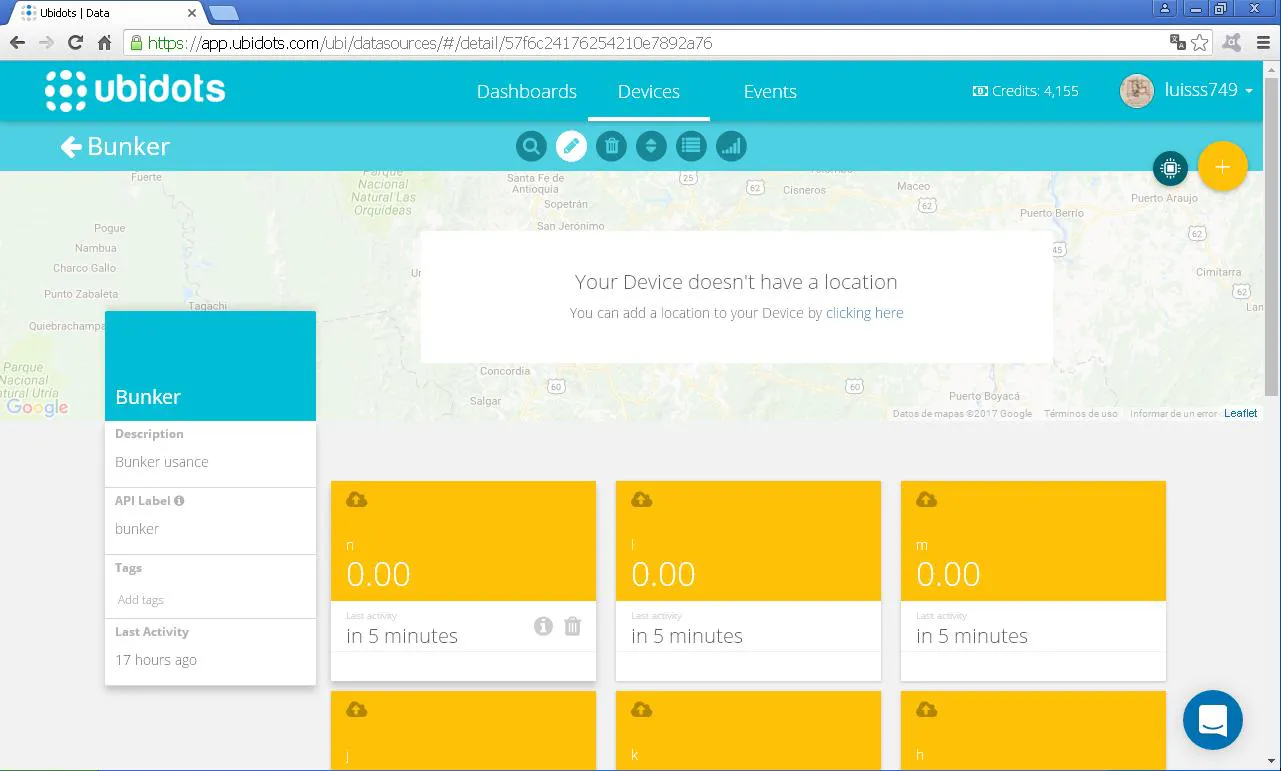### ..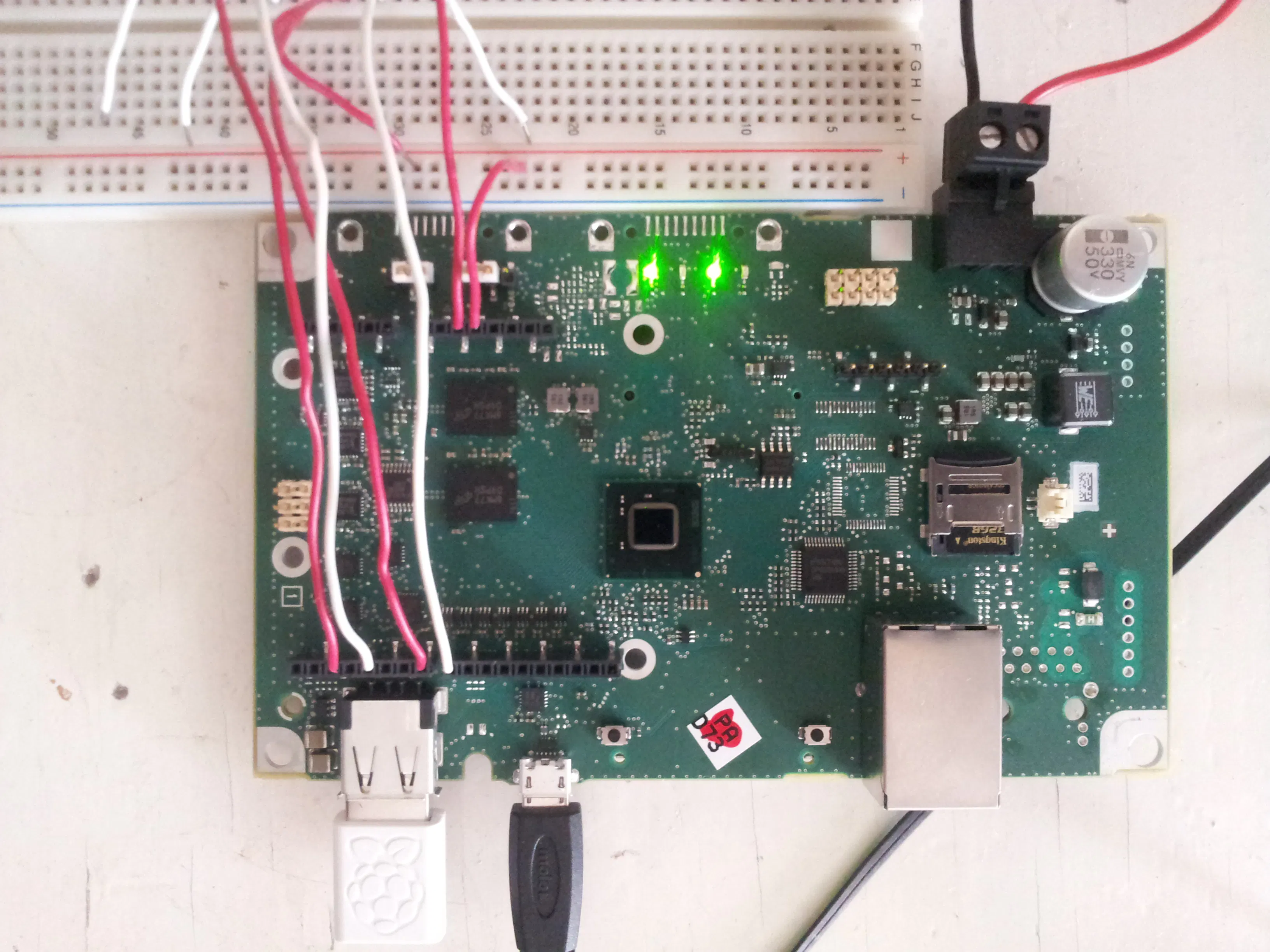### Ubidots results

Here the .csv file generated from the dashboard of ubidots, here you only need to make the sum of each column and divide the result between 100 and with this we will get the amount in minutes of operation time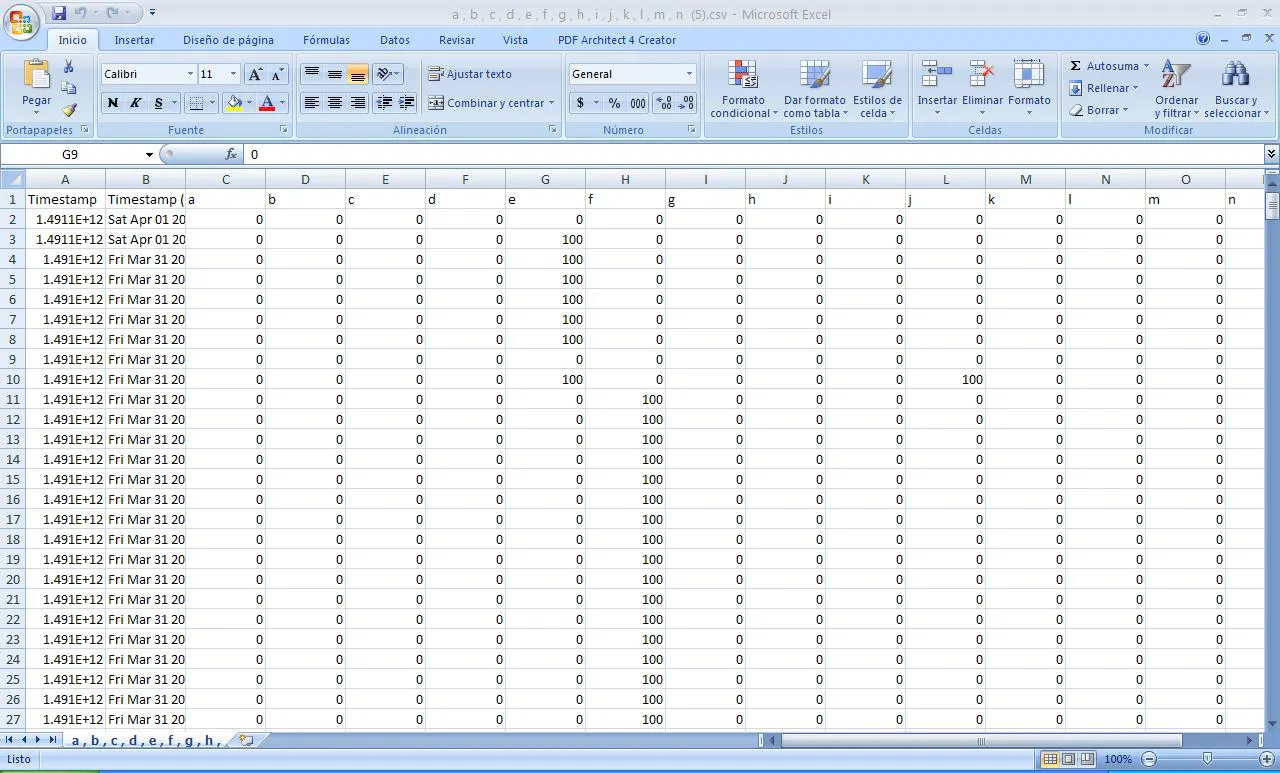## Code

### Industrian Energy Consumption Console Code V1.0

Arduino
This code allows us to send the status (on / off) of the input signals via mqtt using the pubsubclient library, The way to collect the data from ubidots is through a .csv file, to facilitate the interpretation of the data collected, i ve chosen to create a variable for each possible combination of operating states of the system, this gives us a total of 14 variables, i also design this code so that only sends data to ubidots when any value is in the ON state, the time between data sending in on state is 1 min which gives us the resolution enough for our application, when a value returns to OFF state the code connects again to ubidots and changes the state of the variables to zero, although there are many variables the advantage of doing it this way is that we do not need to read all the columns of the inputs in the csv file at the same time to find the mode of operation as would be the case if we only had one variable for each input; To count the total time that a mode of operation has been ON we only need to make the sum of each column separately and dividing by 100 we will have the amount in minutes.
The structure of the code is as follows:
1. Connect AP.
3. Compare inputs and write variables
4. IF any data is different from zero:
A) assembling the data group
B) make connection mqtt
C) send data group
D) disconnect mqtt
C) wait a minute
D) put variable sw = 1
ELSE
IF sw = 1
A) assembling data group with variables = 0
B) make connection mqtt
C) send data group
D) disconnect mqtt
E) put variable sw = 0
```#include <WiFi.h>
#include <PubSubClient.h>

// These are the variables you will want to change based on your IOT data streaming account / provider
#define TOPIC "/v1.6/devices/YOURDEVICELABEL"
#define TOKEN "YOURUBIDOTSTOKEN"

char ssid[] = "YOURNETWORKSSID";
int status = WL_IDLE_STATUS;
char payload;  // Reserve a char to store the Ubidots data. Account for 60 bytes per variable.
char le;
char mqttBroker[] = "things.ubidots.com";
String response;
WiFiClient c;
PubSubClient client(c);

//DEFINING INPUT PINS
int PB1 = 2;
int PB2 = 4;
int PB3 = 7;
int PB4 = 8;

int A = 0;
int B = 0;
int C = 0;
int D = 0;
int E = 0;
int F = 0;
int G = 0;
int H = 0;
int I = 0;
int J = 0;
int K = 0;
int L = 0;
int M = 0;
int N = 0;
int sw = 0;

void setup()
{
system("ifup wlan0");
delay(1000);
Serial.begin(9600);

while (status != WL_CONNECTED)
{
Serial.print("Attempting to connect to SSID: ");
Serial.print(ssid);
status = WiFi.begin(ssid, pass);
delay(10000);
}
Serial.println("Wifi connected!");
client.setServer(mqttBroker, 1883);

pinMode(PB1, INPUT);
pinMode(PB2, INPUT);
pinMode(PB3, INPUT);
pinMode(PB4, INPUT);

}

void loop()
{

// COMPARING INPUTS AND WRITING VARIABLES
if (TO01 ==  1 && TO02 == 1 && TO03 == 1 && Llenado == 1)
{
A = 100;
}
else
{
A = 0;
}

if (TO01 == 1 && A == 0 && F == 0 && G == 0 && I == 0 && L == 0 && M == 0)
{
B = 100;
}
else
{
B = 0;
}
if (TO02 == 1 && A == 0 && F == 0 && H == 0 && J == 0 && L == 0 && N == 0)
{
C = 100;
}
else
{
C = 0;
}
if (TO03 == 1 && A == 0 && G == 0 && H == 0 && K == 0 && M == 0 && N == 0)
{
D = 100;
}
else
{
D = 0;
}
if (Llenado == 1 && A == 0 && I == 0 && J == 0 && K == 0 && L == 0 && M == 0 && N == 0)
{
E = 100;
}
else
{
E = 0;
}
if (TO01 == 1 && TO02 == 1 && A == 0 && L == 0)
{
F = 100;
}
else
{
F = 0;
}
if (TO01 == 1 && TO03 == 1 && A == 0 && M == 0)
{
G = 100;
}
else
{
G = 0;
}
if (TO02 == 1 && TO03 == 1 && A == 0 && N == 0)
{
H = 100;
}
else
{
H = 0;
}
if (TO01 == 1 && Llenado == 1 && A == 0 && L == 0 && M == 0)
{
I = 100;
}
else
{
I = 0;
}
if (TO02 == 1 && Llenado == 1 && A == 0 && L == 0 && N == 0)
{
J = 100;
}
else
{
J = 0;
}
if (TO03 == 1 && Llenado == 1 && A == 0 && M == 0 && N == 0)
{
K = 100;
}
else
{
K = 0;
}
if (TO01 == 1 && TO02 == 1 && Llenado == 1 && A == 0)
{
L = 100;
}
else
{
L = 0;
}
if (TO01 == 1 && TO03 == 1 && Llenado == 1 && A == 0)
{
M = 100;
}
else
{
M = 0;
}
if (TO02 == 1 && TO03 == 1 && Llenado == 1 && A == 0)
{
N = 100;
}
else
{
N = 0;
}

//SENDING DATA (IF DATA != 0)
if (A || B || C || D || E || F || G || H || I || J || K || L || M || N == 100) {

while (!client.connected()) {
Serial.print("Attempting MQTT connection...");
// Attempt to connect
Serial.println("connected");
}
else {
Serial.print("failed, rc=");
Serial.print(client.state());
Serial.println(" try again in 5 seconds");
// Wait 5 seconds before retrying
delay(5000);
}
}
client.disconnect();

delay(60000);
sw = 1;
}

//SENDING DATA ZERO ONLY AFTER A CHANGE OF 100 TO 0 OF ANY VARIABLE

else{

if (sw == 1){

while (!client.connected()) {
Serial.print("Attempting MQTT connection...");
// Attempt to connect
Serial.println("connected");
}
else {
Serial.print("failed, rc=");
Serial.print(client.state());
Serial.println(" try again in 5 seconds");
// Wait 5 seconds before retrying
delay(5000);
}
}
client.disconnect();
sw = 0;
delay (1000);
}
}
}
```

## Credits

### Luis Salazar

2 projects • 5 followers
Mechanical Engineer Electronics hobbyst